# How to count the number of words in a range of cells in Excel

In the previous post, we talked that how to count the number of words in a single cell. And this post explains that how to count the number of words in a range of cells using excel formula. And how to count the total words in a range of cell with VBA Code.

## Count the number of words in a range of cells

If you want to count the number of words in a range of cell, you need to use a combination of the SUMPRODUCT function, the IF function, the LEN function, the TRIM function and the SUBSTITUTE function to create an excel formula.

You need to use the SUBSTITUTE function to remove all spaces from the text for each cell in the range, then using LEN function to calculate the length of the text without spaces. The returned number is subtracted from the length of the text string with space characters to get the number of spaces in the text. And then add 1 to get the number of words in the range.

Assuming that you want to count the number of words in the range B1:B3, you can write down an excel array formula as follows:

`=SUMPRODUCT(IF(LEN(TRIM(B1:B3))=0, 0, LEN(TRIM(B1:B3))-LEN(SUBSTITUTE(B1:B3," ", ""))+1))`

Let’s see how this formula works:

=LEN(TRIM(B1:B3))-LEN(SUBSTITUTE(B1:B3,” “, “”))+1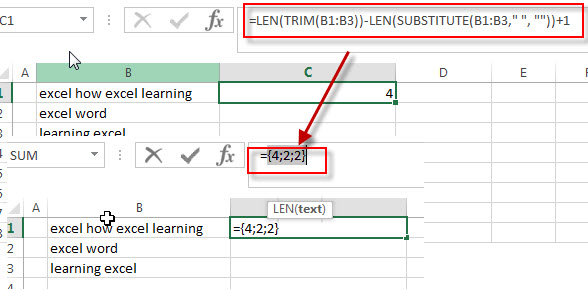This formula returns an array result that contains a number of words for each cell in the range. The array result is like this: {4;2;2}, then it goes into the IF function as its argument.

=IF(LEN(TRIM(B1:B3))=0, 0, LEN(TRIM(B1:B3))-LEN(SUBSTITUTE(B1:B3,” “, “”))+1)

The IF function will check if it is an empty cell, if TRUE, then returns 0, otherwise, returns the number of words in the text in cell.

=SUMPRODUCT()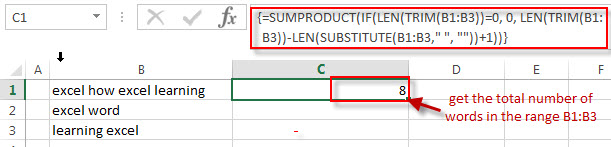This formula will sum all items in that array result that returned by the IF formula to get the total number of words in the range B1:B3.

## Count the number of words in a range of cells with VBA Code

You can also create a new user defined function to count the number of words in a range in Excel VBA:

1# click on “Visual Basic” command under DEVELOPER Tab.2# then the “Visual Basic Editor” window will appear.

3# click “Insert” ->”Module” to create a new module4# paste the below VBA code into the code window. Then clicking “Save” button.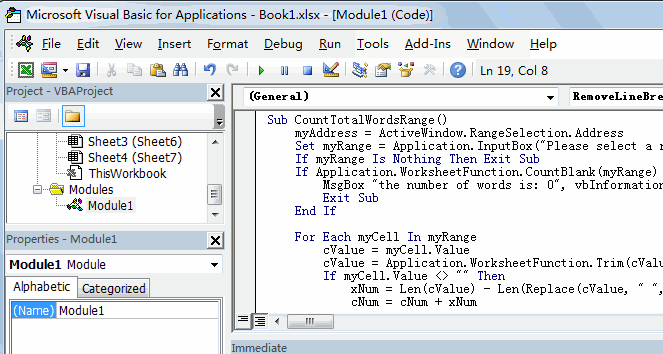```Sub CountTotalWordsRange()
Set myRange = Application.InputBox("Please select a range that you want to count words:", "CountTotalWordsRange", myAddress, , , , , 8)
If myRange Is Nothing Then Exit Sub
If Application.WorksheetFunction.CountBlank(myRange) = myRange.Count Then
MsgBox "the number of words is: 0", vbInformation, "CountTotalWordsRange"
Exit Sub
End If

For Each myCell In myRange
cValue = myCell.Value
cValue = Application.WorksheetFunction.Trim(cValue)
If myCell.Value <> "" Then
xNum = Len(cValue) - Len(Replace(cValue, " ", "")) + 1
cNum = cNum + xNum
End If
Next myCell
MsgBox "the number of words Is: " & Format(cNum, "#,##0"), vbOKOnly, "CountTotalWordsRange"
End Sub```

5# back to the current worksheet, then run the above excel macro, then select a range that you want to count the number of words.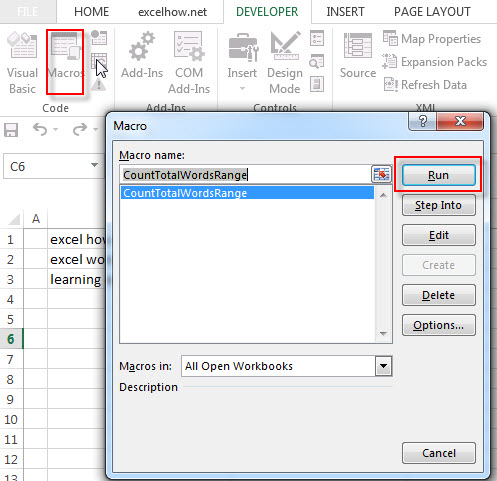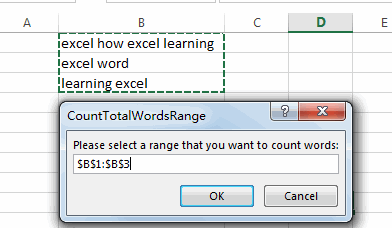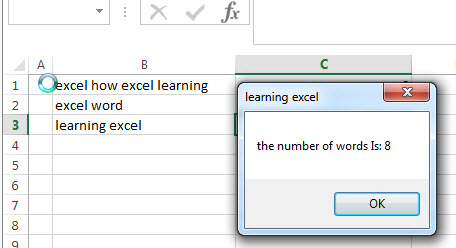### Related Formulas

• count specific words in a cell or a range
If you want to count the number of a specific word in a single cell, you need to use the SUBSTITUTE function to remove all that certain word in text string, then using LEN function to calculate the length of the substring that without that specific word.…
• Count the number of words in a cell
If you want to count the number of words in a single cell, you can create an excel formula based on the IF function, the LEN function, the TRIM function and the SUBSTITUTE function. ..
• Extract word that starting with a specific character
Assuming that you have a text string that contains email address in Cell B1, and if you want to extract word that begins with a specific character “@” sign, you can use a combination with the TRIM function, the LEFT function, the SUBSTITUTE function, the MID function, the FIND function, the LEN function and the REPT function to create an excel formula.…

### Related Functions

• Excel SUMPRODUCT function
The Excel SUMPRODUCT function multiplies corresponding components in the given one or more arrays or ranges, and returns the sum of those products.The syntax of the SUMPRODUCT function is as below:= SUMPRODUCT (array1,[array2],…)…
• Excel Substitute function
The Excel SUBSTITUTE function replaces a new text string for an old text string in a text string.The syntax of the SUBSTITUTE function is as below:= SUBSTITUTE  (text, old_text, new_text,[instance_num])….
• Excel IF function
The Excel IF function perform a logical test to return one value if the condition is TRUE and return another value if the condition is FALSE. The IF function is a build-in function in Microsoft Excel and it is categorized as a Logical Function.The syntax of the IF function is as below:= IF (condition, [true_value], [false_value])….
• Excel LEN function
The Excel LEN function returns the length of a text string (the number of characters in a text string).The syntax of the LEN function is as below:= LEN(text)…
• Excel TRIM function
The Excel TRIM function removes all spaces from text string except for single spaces between words. The syntax of the TRIM function is as below:=TRIM(text)…